Home MATHEMATICS TOPIC 4: UNITS | MATHEMATICS FORM 1

# TOPIC 4: UNITS | MATHEMATICS FORM 1

143## TOPIC 4: UNITS

A unit – is defined as a symbol or sign which is assigned to a number to describe a kind of measurement made

#### Units of Length

Conversion of One Unit of Length to Another

Convert one unit of length to another

The conversion of one unit to another is done by considering the arrangement below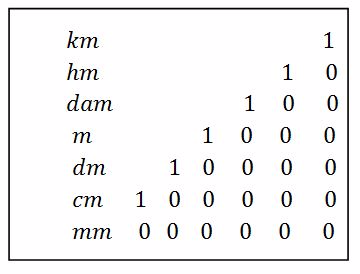Example, from the above we get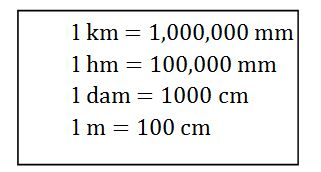Computations on Metric Units of Length

Perform computations on metric units of length

Example 1

Convert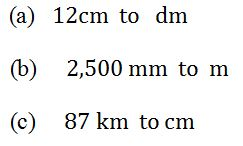Solution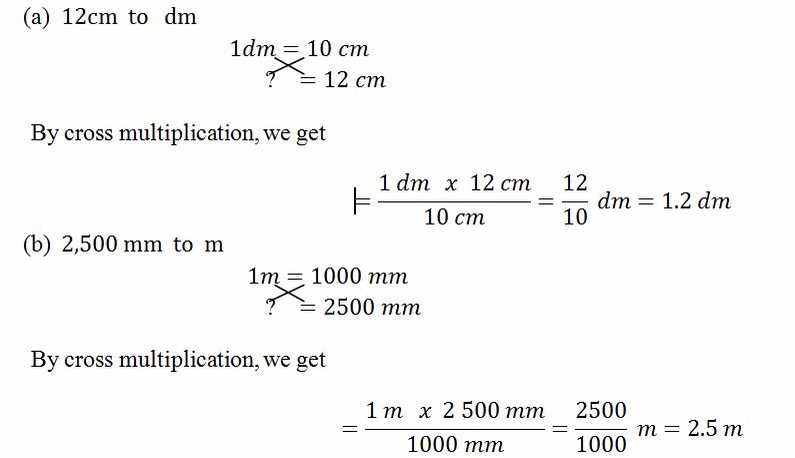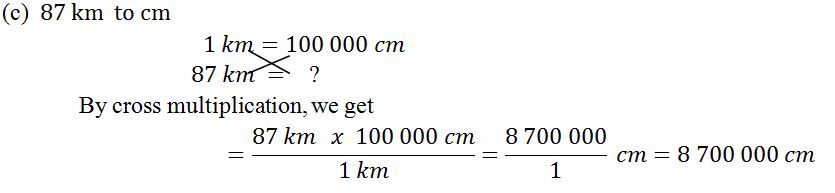#### Unit of Mass

Convertion of One Unit of Mass to Another

Convert one unit of mass to another

The conversion of one unit to another is done by considering the arrangement below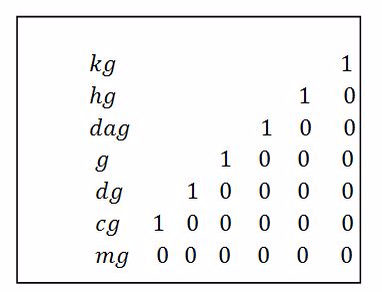Example, from the above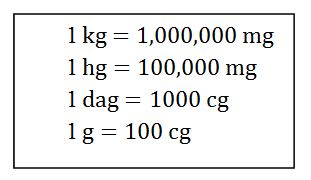The conversion of​​ tonne to other units is done converting it to​​ kilogram first and then from kilogram to the required unit.

1 tonne = 1000kg

Computation on Metric Units of Mass

Perform computation on metric units of mass

Example 2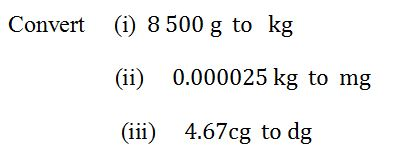Solution